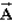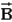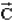# College Physics Study Set 7

Physics & Astronomy

## Quiz 3 : Vectors and Two-Dimensional MotionStudy FlashcardsLooking for Introductory Physics Homework Help?

## Quiz 3 :Vectors and Two-Dimensional Motion

Question TypeThe first displacement is 6 m and the second displacement is 3 m.They cannot add together to give a total displacement of:
Free
Multiple Choice

A

Tags
Choose question tagVectorpoints north and vectorpoints east.If=-, then vectorpoints:
Free
Multiple Choice

B

Tags
Choose question tagIn a 2-dimensional Cartesian coordinate system the y-component of a given vector is equal to that vector's magnitude multiplied by which trigonometric function, with respect to the angle between vector and y-axis?
Free
Multiple Choice

B

Tags
Choose question tagWhich of the following is an example of a vector quantity?
Multiple Choice
Tags
Choose question tagA taxicab moves five blocks due north, five blocks due east, and another two blocks due north.Assume all blocks are of equal size.What is the magnitude of the taxi's displacement, start to finish?
Multiple Choice
Tags
Choose question tagWhen three vectors are added graphically and form a closed triangle, the largest enclosed angle between any two of the vectors cannot be greater than:
Multiple Choice
Tags
Choose question tagA student adds two vectors with magnitudes of 200 and 40.Which one of the following is the only possible choice for the magnitude of the resultant?
Multiple Choice
Tags
Choose question tagThe following force vectors act on an object: i) 50.0 newtons at 45.0 north of east and ii) 25.0 newtons at 30.0 south of east.Which of the following represents the magnitude of the resultant and its angle relative to the easterly direction?
Multiple Choice
Tags
Choose question tagA car is initially moving at 20 m/s east and a little while later it is moving at 10 m/s north.Which of the following best describes the orientation of the average acceleration during this time interval?
Multiple Choice
Tags
Choose question tagWhen we add a displacement vector to another displacement vector, the result is:
Multiple Choice
Tags
Choose question tagIn a 2-dimensional Cartesian coordinate system the x-component of a given vector is equal to that vector's magnitude multiplied by which trigonometric function, with respect to the angle between vector and x-axis?
Multiple Choice
Tags
Choose question tagA hiker walks 200 m west and then walks 100 m north.In what direction is her resulting displacement?
Multiple Choice
Tags
Choose question tagAn object moves at a constant velocity of 11 m/s to the southwest for an interval of 20 s.Halfway through this interval, what is the magnitude of its instantaneous velocity?
Multiple Choice
Tags
Choose question tagIn a 2-dimensional Cartesian system, the x-component of a vector is known, and the angle between vector and x-axis is known.Which operation is used to calculate the magnitude of the vector? (taken with respect to the x-component)
Multiple Choice
Tags
Choose question tagAn object, initially moving in the negative x-direction, is subjected to a change in velocity in the positive y-direction.If the resulting velocity vector is drawn from the origin, into which quadrant does this vector point?
Multiple Choice
Tags
Choose question tagA runner circles a track of radius 100 m one time in 100 s at a constant rate.The greatest change in his velocity from his starting velocity:
Multiple Choice
Tags
Choose question tagWhen we subtract a velocity vector from another velocity vector, the result is:
Multiple Choice
Tags
Choose question tagFind the resultant of the following two vectors: i) 50 units due east and ii) 100 units 30 north of west.
Multiple Choice
Tags
Choose question tagWhich type of quantity is characterized by both magnitude and direction?
Multiple ChoiceVectoris 3 m long and vectoris 4 m long.The length of the sum of the vectors must be: